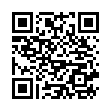# North Coast Synthesis Ltd.

## G♭♭5+2+4Choose audio sample set:
MSK 007 Leapfrog VCF (patch; see in online store)
MSK 009 Coiler VCF (patch; see in online store)

Equivalent chord symbols: A♭9-5+♯2, F♯♭5+2+4, F♯♭5+4+9, G♭♭5+4+9, F♯♭5+2+11, G♭♭5+2+11.

Notes in this chord: G♭, A♭, B♭, C♭, D♭♭. Integer notation: {0, 6, 8, 10, 11}.

Nearby chords (one less note): G♭4+2, G♭♭5+2, G♭♭5+4, B♭2+7+♯1, G♭sus4♭5+2.

Nearby chords (one more note): A♭9+♯2, G♭11♭5, G♭M11♭5, B♭9♯5+♯1, G♭+2+4+♯4, G♭♭5+2+4+♯1, G♭dim+2+4+♯3.

Parallel chords (same structure, different root): C♭♭5+2+4, D♭♭5+2+4, E♭♭5+2+4, F♭♭5+2+4, A♭♭5+2+4, B♭♭5+2+4, C♯♭5+2+4, D♯♭5+2+4, E♯♭5+2+4, F♯♭5+2+4, G♯♭5+2+4, A♯♭5+2+4, B♯♭5+2+4.

Experimental fretting charts for guitar standard EADGBE tuning (change tuning or instrument):